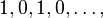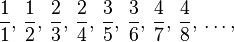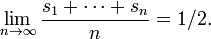What is the sum of the infinite sequence 1, -1, 1, -1, 1…..?

This is a really interesting puzzle to study – which fits very well when studying geometric series, proof and the history of maths.

The two most intuitive answers are either that it has no sum or that it sums to zero.  If you group the pattern into pairs, then each pair (1, -1) = 0.  However if you group the pattern by first leaving the 1, then grouping pairs of (-1,1) you would end up with a sum of 1.

Firstly it’s worth seeing why we shouldn’t just use our formula for a geometric series:with r as the multiplicative constant of -1.  This formula requires that the absolute value of r is less than 1 – otherwise the series will not converge.

The series 1,-1,1,-1…. is called Grandi’s series – after a 17th century Italian mathematician (pictured) – and sparked a few hundred years worth of heated mathematical debate as to what the correct summation was.Using the Cesaro method (explanation pasted from here )

If an = (−1)n+1 for n ≥ 1. That is, {an} is the sequenceThen the sequence of partial sums {sn} isso whilst the series not converge, if we calculate the terms of the sequence {(s1 + … + sn)/n} we get:so thatSo, using different methods we have shown that this series “should” have a summation of 0 (grouping in pairs), or that it “should” have a sum of 1 (grouping in pairs after the first 1), or that it “should” have no sum as it simply oscillates, or that it “should”  have a Cesaro sum of 1/2 – no wonder it caused so much consternation amongst mathematicians!

This approach can be extended to the complex series,$1 + i + i^2 + i^3 + i^4 + i^5 + \cdots$ which is looked at in the blog  God Plays Dice

This is a really great example of how different proofs can sometimes lead to different (and unexpected) results. What does this say about the nature of proof?